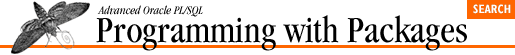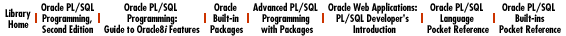home | O'Reilly's CD bookshelfs | FreeBSD | Linux | Cisco | Cisco Exam

### 5.27 PLVtmr: Program Performance Analyzer

The PLVtmr (PL/Vision TiMeR) package allows you to measure the elapsed time of PL/SQL code; it provides a programmatic layter around the GET_TIME function of Oracle's DBMS_UTILITY package. See Chapter 14, PLVtmr: Analyzing Program Performance for details.

### 5.27.1 Control execution of timings

``` PROCEDURE turn_on;```

Enables the timing package. All PLVtmr programs will execute as requested.

``` PROCEDURE turn_off;```

Disables PLVtmr. Calls to timing programs will be ignored.

### 5.27.2 Setting the repetition value

``` PROCEDURE set_repeats (repeats_in IN NUMBER);```

Sets the number of repetitions for all timing loops implemented inside the PLVtmr package ( calibrate , func , currsucc , currfail ).

``` FUNCTION repeats RETURN NUMBER;```

Returns the current repetition value.

### 5.27.3 Setting the factoring value

``` PROCEDURE set_factor (factor_in IN NUMBER);```

Sets the number of iterations when calling PLVtmr in a loop. This value allows PLVtmr to show total and individual elapsed times.

``` FUNCTION factor RETURN NUMBER;```

Returns the current number of iterations.

### 5.27.4 Capturing the current timestamp

``` PROCEDURE capture (context_in IN VARCHAR2 := NULL);```

Calls capture to capture the current timestamp (usually the start time of an elapsed time calculation). You can provide an optional context for the timing.

### 5.27.5 Retrieving and displaying elapsed time

``` FUNCTION elapsed RETURN NUMBER;```

Returns the number of hundredths of seconds elapsed since last call to capture .

``` FUNCTION elapsed_message```
``` (prefix_in IN VARCHAR2 := NULL,```
``` adjust_in IN NUMBER := 0,```
``` reset_in IN BOOLEAN := TRUE,```
``` reset_context_in IN VARCHAR2 := NULL)```
``` RETURN VARCHAR2;```

Returns a standard message format around the value returned by elapsed .

``` PROCEDURE show_elapsed```
``` (prefix_in IN VARCHAR2 := NULL,```
``` adjust_in IN NUMBER := 0,```
``` reset_in IN BOOLEAN := TRUE);```

Displays the elapsed time message returned by elapsed_message .

### 5.27.6 Calibration and timing scripts

PLVtmr offers a set of predefined scripts and calibration programs to test comparative performances. You might find these particular programs useful; you might simply follow their example to construct your own.

``` PROCEDURE calibrate;```

Calculates a base timing  -- the amount of time required to execute the NULL statement the current number of repetitions (set through set_repetitions ).

``` FUNCTION base_timing RETURN NUMBER;```

Returns the current base timing.

``` PROCEDURE func;```

Calculates the overhead of a function call.

``` PROCEDURE cursucc;```

Compares the performance of implicit and explicit cursors when retrieving a row successfully.

``` PROCEDURE curfail;```

Compares the performance of implicit and explicit cursors when failing to retrieve a row.5.26 PLVtkn: Token Table Interface5.28 PLVtrc: Trace Facility